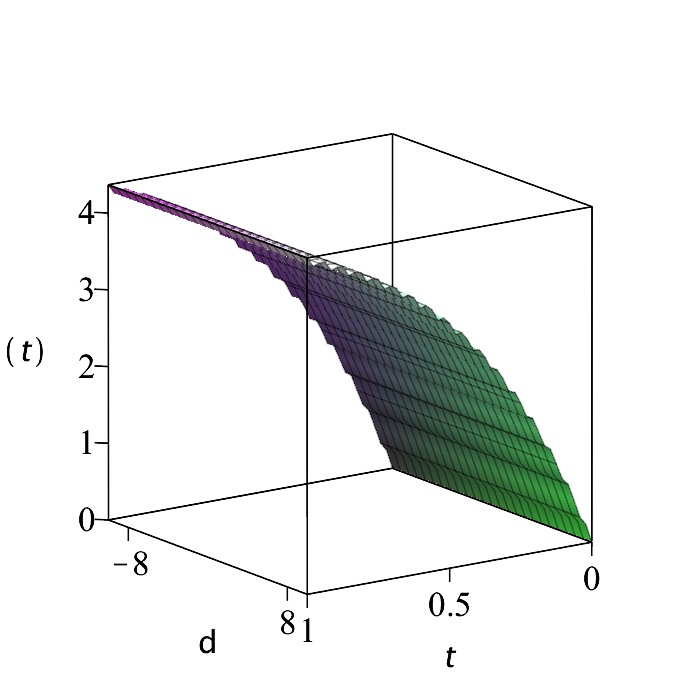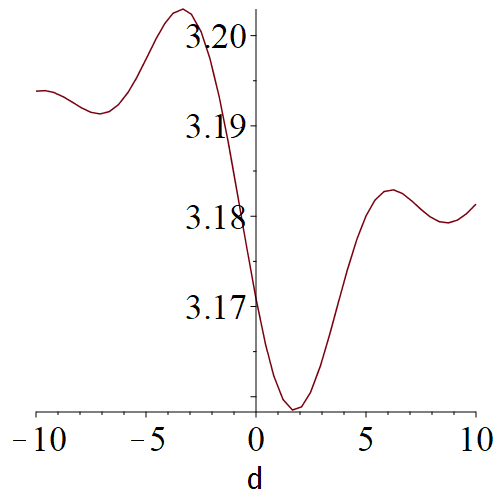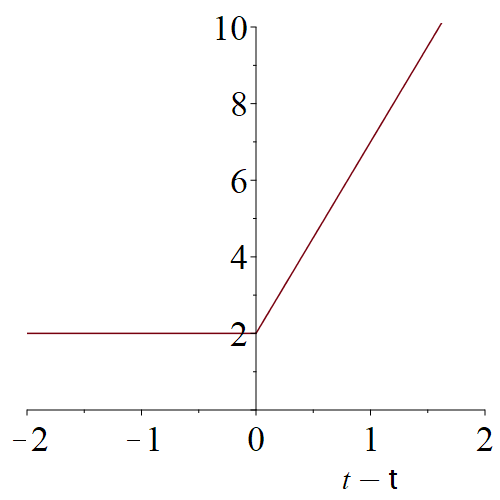Carl Love

## 24 Badges

8 years, 246 days
Natick, Massachusetts, United States
My name was formerly Carl Devore.

## Array plot not related to graph theory...

I don't have Maple 2021 to check this, but I suspect that the problem that you report applies to any array plot. To test my hypothesis, try exporting this 2x2 array of plots to latex:

plots:-display(plot~(<x, x^2; x^3, x^4>));

## Plots using a dsolve parameter as indepe...

I think that @mmcdara has made this seem more "complex" than it really is. Here is how to do the 3D plot that you want:

```restart
:
par:= {
phi= 0, lambda= 0.1, N= 5,
M= sqrt(N*(N+1))*exp(I*phi), omegap= 10
}:
var:= {n,u,z}(t):
dsys:= {
diff(n(t),t) =
-2*(n(t)-N)+(u(t)-M)*exp(-2*I*omegap*t/lambda) +
((z(t)-conjugate(M))*exp(2*I*omegap*t/lambda)),
diff(u(t),t) =
-2*(1-I*delta)*u(t) + 2*(n(t)-N)*exp(2*I*omegap*t/lambda)+2*M,
diff(z(t),t) =
-2*(1+I*delta)*z(t) +
2*(n(t)-N)*exp(-2*I*omegap*t/lambda)+2*conjugate(M)
}:
res1:= dsolve(
eval[recurse](dsys, par) union (eval(var, t= 0)=~0),
numeric, parameters= [delta], output= listprocedure
):

#Function to be plotted, and a helper function to speed things up:
Ndelta:= proc(delta::numeric)
option remember;
res1(parameters= [delta]);
#Return the instantiated procedure:
sscanf(sprintf("%m", eval(eval(n(':-t'), res1))), "%m")[]
end proc
:
N:= (t, delta)->
`if`([args]::list(numeric), Ndelta(delta)(t), 'procname'(args))
:
P3:= CodeTools:-Usage(
plot3d(
N(t, delta), t= 0..1, delta= -10..10, axes= boxed,
tickmarks= [3, [-8, 8], 5],
titlefont= [Helvetica, roman, 18],
labels= [t, delta, n(t)],
labelfont= [Helvetica, roman, 24]
)
);
memory used=8.47GiB, alloc change=0 bytes,
cpu time=84.97s, real time=78.92s, gc time=14.45s

#Show delta variation for fixed t:
P2:= CodeTools:-Usage(
plot(N(.5, delta), delta= -10..10, numpoints= 49, adaptive= false)
);
memory used=4.69GiB, alloc change=48.00MiB,
cpu time=50.72s, real time=47.10s, gc time=8.64s

```At this scale, the variation with respect to delta is visually insignificant compared to the variation with respect to t. You can see it by using a much smaller t range or, perhaps, a much larger delta range.## Replace t-tau (automatically)...

I'm not sure what you mean by "system of equation". What you show is a single piecewise expression in two variables. You got an error because there are two independent free variables. In this case, they can be easily replaced by a single variable. So, perhaps the below plot is what you want. Let me know.

pw:= piecewise(t-tau <= 0, 2, 2+5*(t-tau)):
plot(simplify(pw, {t-tau = s}), s= -2..2, 0..10, labels= [t-tau, ``]);I'm not pleased about the default font choice for the tau on the axis label! I suspect that I could easily fix that if you want. Also, it may appear as a normal Greek tau on your display (not here, but within Maple).

## Rescaling and forced tickmarks...

Here's an example of what @acer meant by "rescaling and forced tickmarks". For use over significantly wider or narrower ranges, the round in procedure ReTick will need to be changed. [Edit: I now see that acer has updated his Answer to provide such an example. His acts after the plot is created, and mine acts before. Both approaches have their pros and cons. In either case, having the z-axis constrained to a "nice" range by view makes things significantly easier.]

```ReTick:= proc(ab::range(realcons), s::positive, n::posint:= 5)
local a:= lhs(ab), h:= (rhs(ab)-a)/n/2, k;
':-tickmarks'=
[seq](round(a+k*h)/s = `if`(k::odd, "", round(a+k*h)), k= 0..2*n)
end proc
:
(sx,sy):= (1,5): #axes scale factors
f:= (x,y)-> abs(Zeta(x+y*I)):
(xr,yr):= (-4..5, -10..40): #axes ranges
plot3d(
f@((x,y)-> (sx*x, sy*y)), map(`/`, xr, sx), map(`/`, yr, sy),
labels= ['x', 'y', ``], view= [DEFAULT, DEFAULT, 0..4],
axis= [ReTick(xr, sx, 4)], axis= [ReTick(yr, sy)],
orientation= [-15, 75, 0], scaling= constrained
);
```